## Microsoft Math Add-in for Microsoft Word 2007/2010

Nov 30, 2007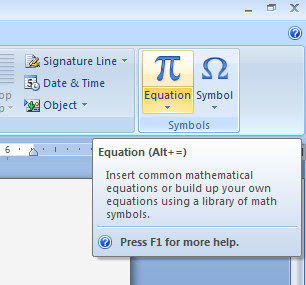If you are a student, then you must know the very powerful Equation function of Microsoft Word 2007. Extremely convenient for writing equations, the only thing it lacks is the ability to do the calculations…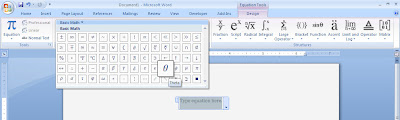Now with Microsoft Math Add-In for Word 2007, you can not only perform calculations but also have a Word equivalent of what the separate application Microsoft Math 2007 (bundled with Microsoft Student) offers.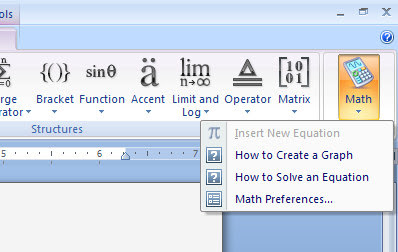The Microsoft Math Add-in for Microsoft Office Word 2007 makes it easy to create graphs, perform calculations, and solve for variables with equations created in Word.
The Microsoft Math Add-in adds computational and graphing capabilities to the Equation Tools Ribbon of Word 2007.

With the Microsoft Math Add-in for Word 2007, you can:
- Plot a function, equation, or inequality in 2-D or 3-D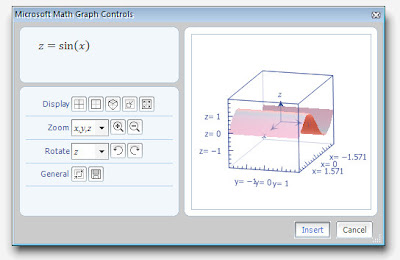- Solve an equation or inequality- Calculate a numerical result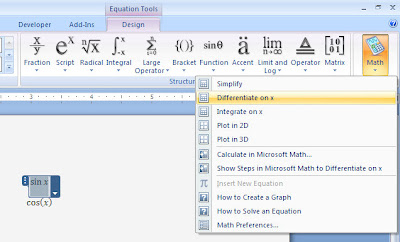- Simplify an algebraic expression
To use the add-in, open Word 2007, type Alt-= to create a RichEdit math object, type an equation or expression, and right-click on the equation to see options for solving and graphing within Word.

Microsoft Math stuck?No… it’s just that you need to specify whether the equation is in radians or degrees & whether you want complex or real numbers.As a side-note, there’s also Equation Wizard that is quite good as Microsoft Math in solving equations…Update:Do you have any suggestions? Ideas? Add your comment. Please don't spam & don't swear! Subscribe to my feed
InF said...

Very very useful! Thanks Carrot! :P

I found a new use for Word now.. Be my calculator! :P

Some html tags like <b>, <i>, <a> are allowed & emos can be used. ;)
Copy your comment before posting in case something fails.
Comments are moderated, may take a while to appear.